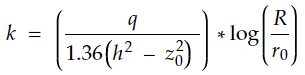top of page
Search
• APSEd

# Soil Permeability Tests | Lab and Field Tests for Permeability

Permeability is a property of a porous medium like soil by virtue of which any fluids are able to flow through that medium. Permeability of soil can be found either in a lab or in field conditions. Important methods of permeability testing, used in both lab and field approaches are discussed further.

### Laboratory Tests to Determine Permeability

Laboratory methods are easier to perform than field methods. There are many laboratory methods to determine permeability as listed below.

2. Falling or variable head permeameter

3. Direct or indirect measurement during odometer test

4. Horizontal capillarity test

Out of all these methods, constant head and variable head permeameter tests are commonly done.

### Field Tests for Determining Permeability

Field tests are required because permeability depends on both the microstructure (like arrangement of soil grains) and the macrostructure (like stratification) of soil. It may also be required in cases where getting a representative sample is difficult. Below listed are the field methods to determine permeability.

1. Pumping out of wells

2. Pumping into wells

The principle in this test is that the hydraulic head causing the flow is maintained as constant. The quantity of water flowing through a soil specimen of known cross-sectional area and length in a given time is measured.

This method is commonly done for relatively previous soil, as the quantity of water flow in an impervious soil is less and may not be measured accurately.

q = Q/t = k * i * A

k = (Q/t) * (1 / (i * A) ), where i = h/L

k = (Q * L) / (t * h * A)

where,

k - Darcy's coefficient of permeability

L and A - length and area of cross-section of soil specimen

h - hydraulic head causing flow

### Falling or Variable Head Permeability Test

In this method, the water level in the stand-pipe falls continuously as water flows through the specimen. Measurement is taken in terms of both head changes and corresponding time changes. For example, the head falls from h0 to h1, corresponding to the elapsed time to and t1. This test can be used for relatively impervious soil.

k = ( (2.303*a*L) / (A* (t1 - t0) ) ) * log(h0/h1)

where,

a - area of cross-section of the standpipe

L and A - length and area of cross-section of the soil sample

h0, h1 - head at time t0 and t1 respectively

Note: In both these tests, observations are to be made only after a steady state of flow is achieved.

### Field Test for Permeability - Pumping Out Test

#### Aquifer

Before getting into the details of the pumping-out test, it is important to know about aquifers. An aquifer is a permeable formation that allows a significant quantity of water to move through it under field conditions. Broadly there are two types of aquifers based on the position of water strata within the soil, namely unconfined aquifer, and confined aquifer.

#### Drawdown Curve

The next term to understand is "drawdown curve". To perform the pumping-out test, a well needs to be penetrated into the soil stratum. The water table in the well will initially remain horizontal. But when water is pumped out from the well, the aquifer gets depleted, and the water table is lowered resulting in a circular depression. This is called the "drawdown curve" or "cone of depression".

In pumping out tests, drawdown corresponding to a steady discharge is observed at a number of observation wells. To establish this steady condition, pumping must be carried out at a uniform rate for an adequate time.

#### Assumptions

• Flow is laminar and Darcy's law is valid

• Flow is horizontal and uniform at all points in the vertical section

• The aquifer is homogeneous with uniform permeability

• Well penetrates the entire thickness of the aquifer

• The natural groundwater regime affecting the aquifer remains constant with time

There are two separate formulas to find out permeability for unconfined and confined aquifers. Illustrations and formulas for both conditions are mentioned below.

Unconfined Aquifers

Below given is the illustration used to derive the formula for permeability in an unconfined aquifer. The formula is derived using Darcy's law.Pumping out Test: Unconfined AquiferFrom finding z1, z2, and r1, r2, from field observations, k can be evaluated. The equation can also be reduced by applying the extreme limits z0 and h at r0 and R as given below.Confined Aquifers

Below given is the illustration used to derive the formula for permeability in a confined aquifer. The formula is derived using Darcy's law.Pumping out Test: Confined AquiferFrom finding d1, d2, and r1, r2, from field observations, k can be evaluated. The equation can also be reduced by applying the extreme limits z0 and h at r0 and R as given below.With these, we come to the end of this blog. As direct permeability measures, there are also indirect measures to find the permeability. If you want to know about the indirect measures of permeability type "Permeability" in the form below and we will cover it in our next blog.

bottom of page